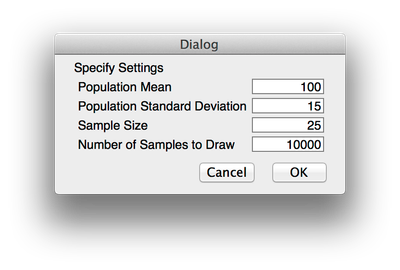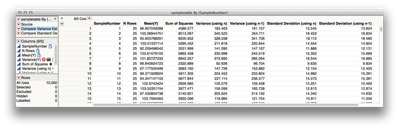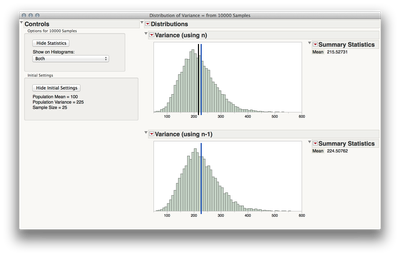julianCommunity Manager

## Simulating the estimation of the population variance (Why n-1?)

Video Demo

The attached script is useful for demonstrating the bias in estimating the population variance/standard deviation without using the corrected sample estimator (with n-1). This script can be used during lecture to demonstrate the concept, or can be given to students to use an an exploratory exercise.

Usage:

1. Run the attached script by pressing Control-R (on the PC), and Command-R (on the Mac).

2. Specify the population parameters, sample size, and number of sample to draw. (10k or more samples is recommended, and should take less than ~3 seconds on most computers)3. The table of sample estimates will be generated, including:

• Sample Number
• Sample Size
• Sums of Squares for each sample
• Variance, estimated using the population form (with N in the denominator)
• Variance, estimated using the sample estimator form (with n-1 in the denominator)
• Standard Deviation, estimated using the population form (with N in the denominator)
• Standard Deviation, estimated using the sample estimator form (with n-1 in the denominator)4. Run the saved scripts in the table to visualize and interact with the observed estimates. In the interface you can:

• Show the population variance/standard deviation on the histogram
• Show the observed mean estimate of the selected statisticFor ideas on using this script, see the Video Demo that I use in my course.

This script was developed in collaboration with mia.stephens. Please post any suggestions for future versions.

We hope this helps with your teaching!

julian

Article Labels
Article Tags
Contributors